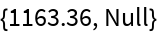#Function Repository Resource:

# TrigDataset

Get trigonometric datasets including closed-form values

Contributed by: Claudio Lobo Chaib Filho
 ResourceFunction["TrigDataset"][f] gets a dataset for the specified trigonometric function f.

## Details and Options

The function f can be any of the following: Sin, Cos or Tan (or "Sine", "Cosine" or "Tangent").
The angles in the generated table are in steps of 3 degrees by default, but it is possible to have closed-form values with different granularity.
ResourceFunction["TrigDataset"] supports the following options:
 "Digits" 2 precision of the "Decimal" result "DegreeStep" 3 step size (in degrees) between rows in the result
The "DegreeStep" option accepts Quantity, either as a degree or as radians. If not specified by Quantity, the default is degree.

## Examples

### Basic Examples

Generate a dataset for the Sin function:

 In:=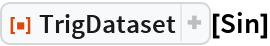Out=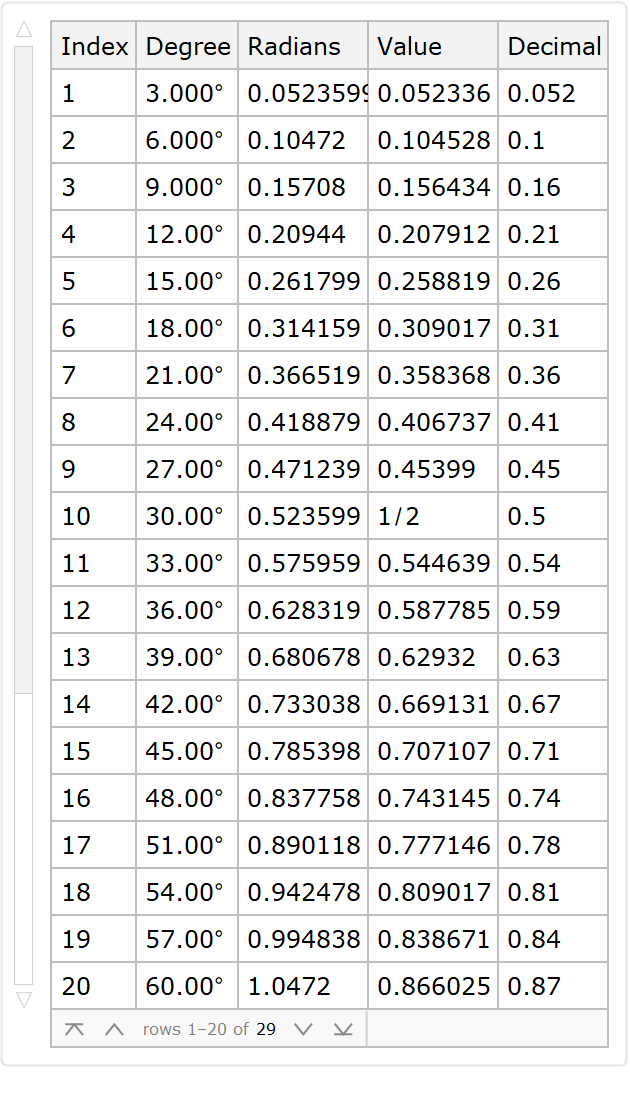### Scope

Generate some trigonometric data:

 In:=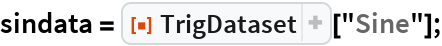Show all information for a specified row:

 In:=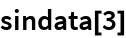Out=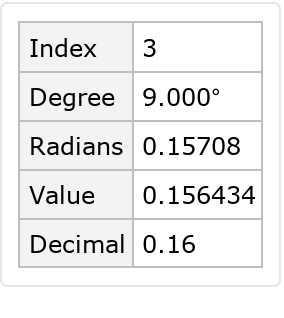Select one of the columns in this row to show the result in standard closed form:

 In:=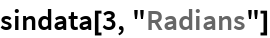Out=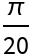Show the entire row outside of the dataset with StandardForm:

 In:=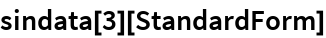Out=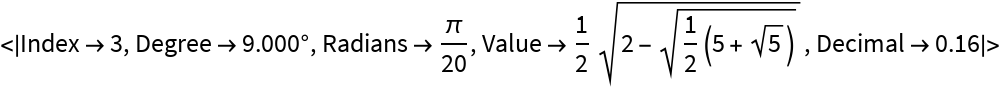### Options

With the "Digits" option, one can get any precision value for the results in the "Decimal" column:

 In:=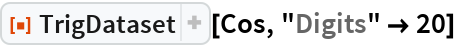Out=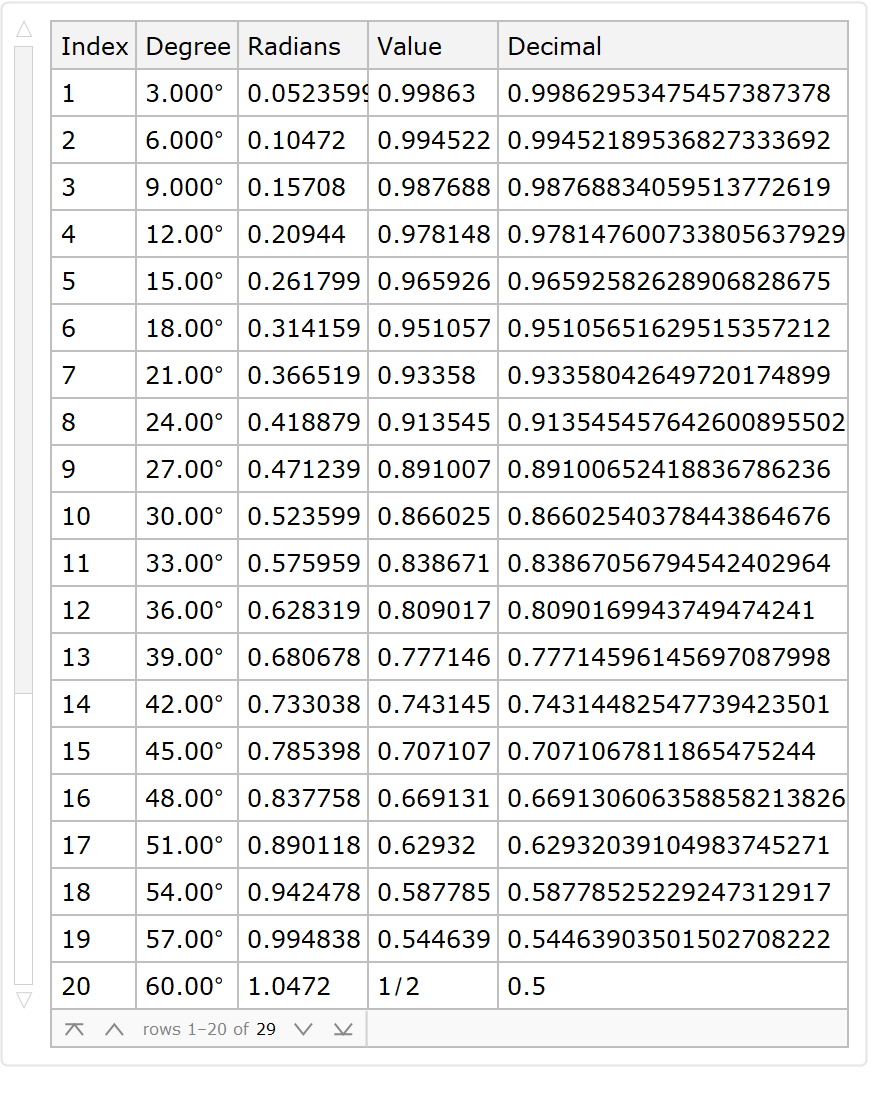Use the option "DegreeStep" to specify the difference between the angle at successive rows in the results. Values which are multiples of 1/8 will give results in terms of radicals:

 In:=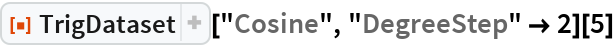Out=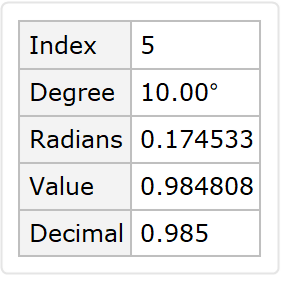Use 12-degree steps:

 In:=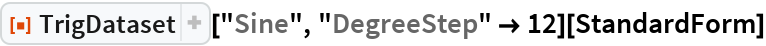Out=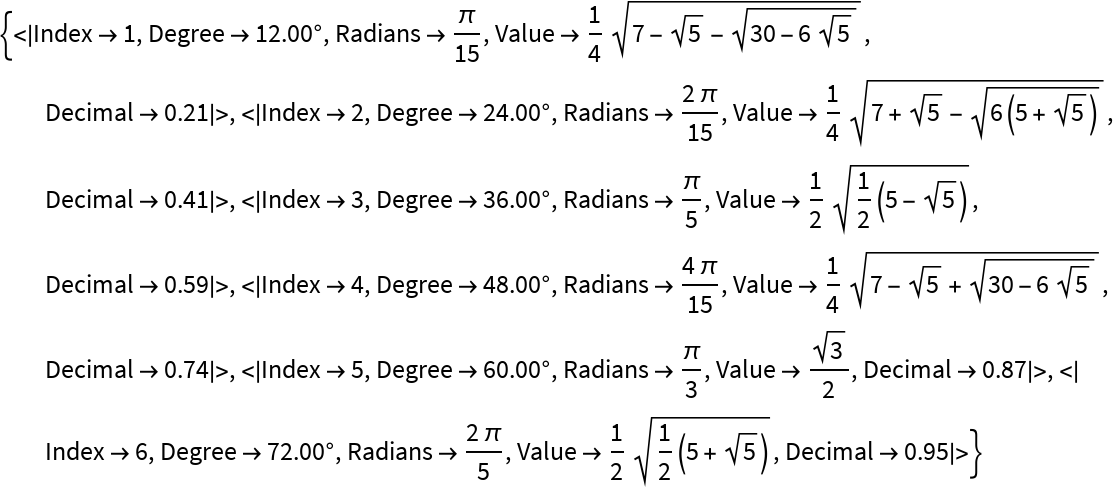Show multiple results simultaneously:

 In:=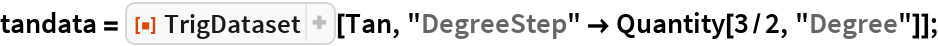In:=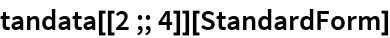Out=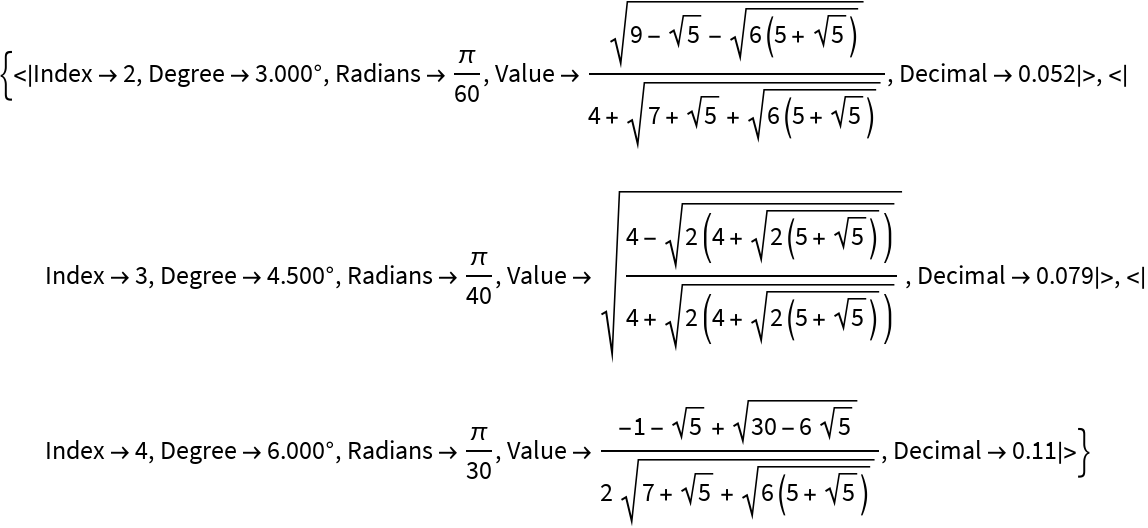For many "DegreeStep" values, one can obtain a radical result using "Value":

 In:=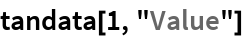Out=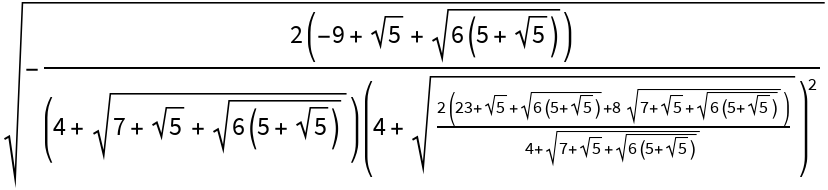Use a Quantity for "DegreeStep"to specify radians:

 In:=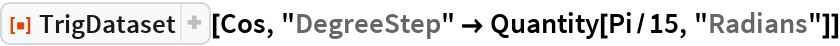Out=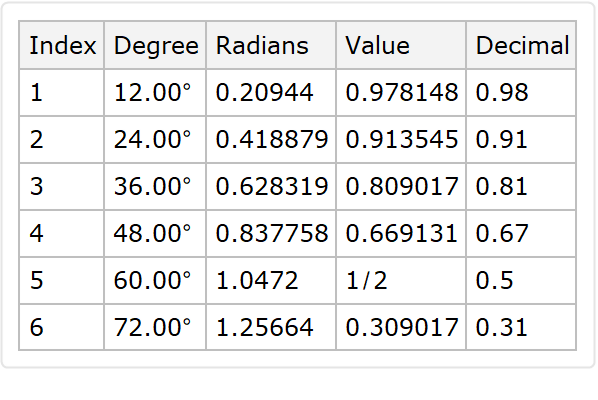In:=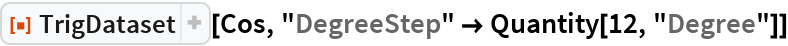Out=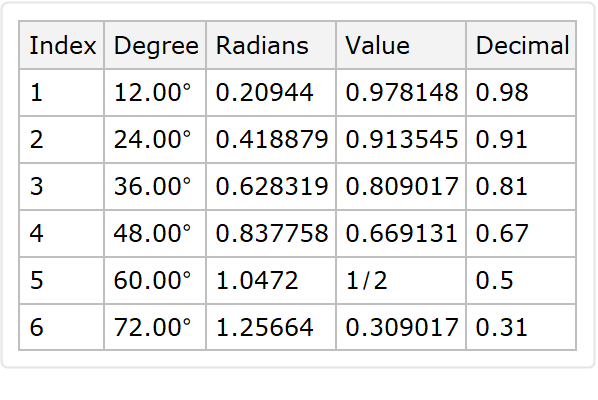### Possible Issues

Sometimes the result of the closed form appears inside the dataset, which is not very useful for copying the result:

 In:=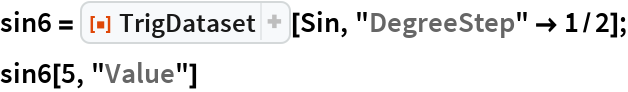Out=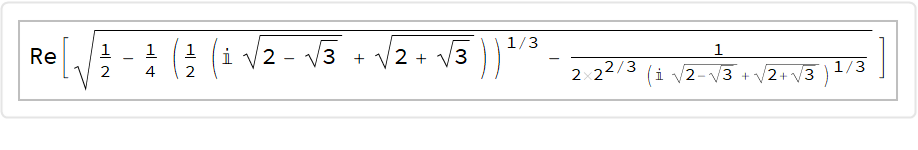Retrieve the StandardForm:

 In:=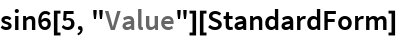Out=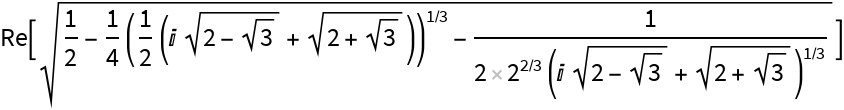The smaller the "DegreeStep", the longer the time to evaluate. Tangent with step 1/2 takes about 10 minutes and step 3/8 takes about 20 minutes:

 In:=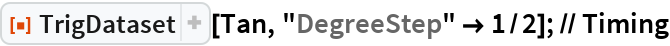Out=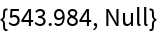In:=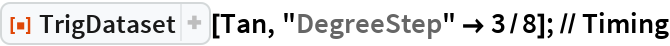Out=